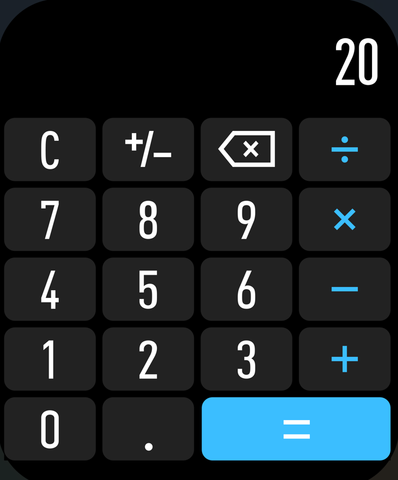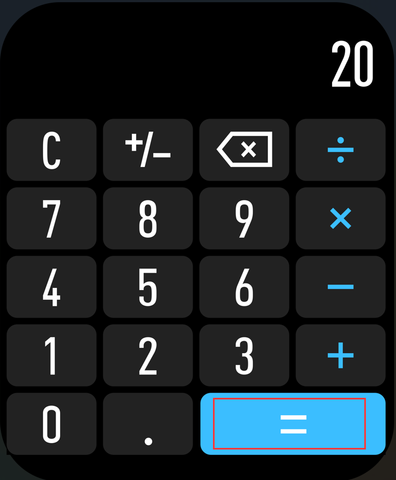# COLMi P68 Calculator Features

The smart watch COLMi P68 has a built-in calculator function, and users can use its calculator function through the following steps:

1. First, swipe from the home screen of the watch to the application list on the left, find and tap the "Calculator" icon.2. After entering the calculator interface, the user can see a numeric keyboard and some basic calculation symbols. Input the numbers and operators to be calculated by clicking the numeric keypad and symbol buttons.3. After the calculation is completed, the user can click the "=" button to display the result.4. If the user wants to perform a new calculation, he can click the "C" button to clear the data in the input box of the calculator.In short, it is very simple to use the calculator function of COLMi P68. Users only need to open the calculator application and enter the corresponding numbers and calculation symbols.